Latest Banking jobs   »   Quantitative Aptitude Quiz For IBPS PO...

# Quantitative Aptitude Quiz For IBPS PO Mains 2022-9th January

Q1. Is A² + B² + C² + D² divisible by 4?
(I) A, B, C and D are consecutive even number.
(II) A, B, C and D are consecutive odd number.
(III) A, B, C and D are consecutive prime numbers.
(a) Either statement ‘I’ alone or ‘II’ alone is sufficient to answer the question
(b) Either statement ‘II’ alone or ‘III’ alone is sufficient to answer the question
(c) Either statement ‘III’ alone or ‘I’ alone is sufficient to answer the question
(d) Any of two statements are sufficient to answer the question
(e) Any one of them is sufficient to answer the question

Directions (2-5): Pie chart given below shows export distribution of 6 countries in percentage in 2017. Bar chart shows the increase in the exports percentage of these 6 respective countries in 2018 as compare to 2017 .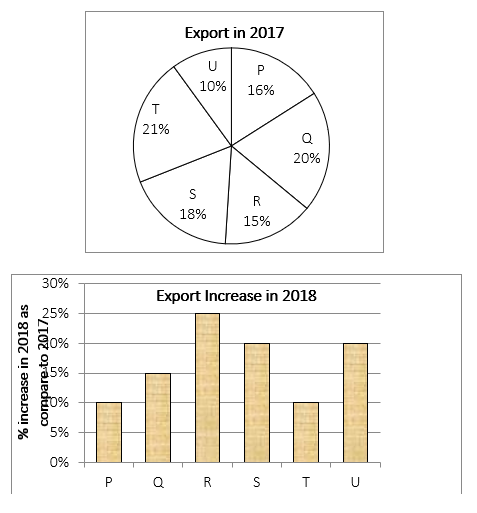Q2. What is the percentage increase in total export in 2018 as compare to 2017?
(a) 15.02%
(b) 14.02%
(c) 16.05%
(d) 100%
(e) None of these

Q3. If total export in 2018 of all countries is Rs. 37136 cr then find the export of country S in 2017.
(a) 5430 cr.
(b) 5160 cr.
(c) 5400 cr.
(d) 5760 cr.
(e) None of these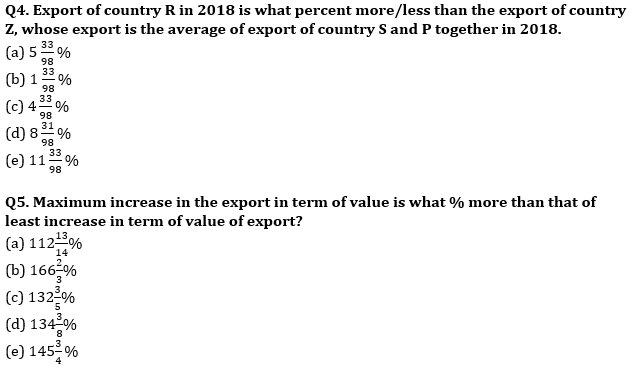Directions (6-10):The graph shows the overall % of interest obtained on principal invested by five persons for different time period. Table below shows the few of details of money invested and obtained by five friends.
NOTE:
‘Amount invested’ means the principal value of a person.
‘Amount obtained’ means the amount which he received after his given time period.
ROI is calculated on per annum basis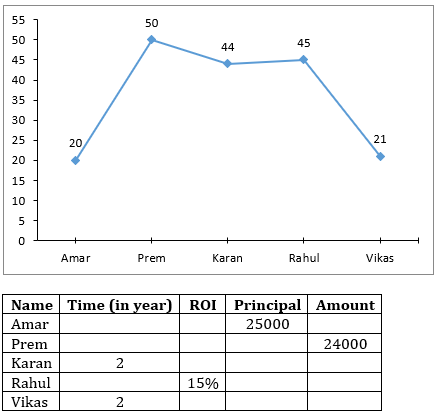Answer the following questions on the basis of information.

Q6. Amount obtained by Vikas after two years is submitted in the bank whose interest rate is same as that of bank of Karan. Find the total amount with him (Vikas) after further two years is what % of his initial principal.
(a) 74.24 %
(b) 37.36%
(c) 92.46%
(d) 174.24%
(e) 167.3%

Q7. If Karan had invested his amount for 1 more year, the amount received by him is Rs. 6336 more than now. Calculate principal invested by him.
(a) Rs. 22,000
(b) Rs. 120000
(c) Rs. 28,800
(d) Rs. 15112
(e) Either Rs. 22,000 or Rs. 28,800

Q8. Ratio of ROI of each year for Amar and Prem is inverse ratio of their amount obtained. Find the ratio of their time (Amar : Prem) for which they invested given that both invested at SI.
(a) 1 : 2
(b) 3 : 4
(c) 4 : 3
(d) 4 : 5
(e) can’t be determined

Q9. Karan invested 33 ⅓% of amount obtained by Amar. Rahul invested 83 ⅓% of amount obtained by Karan. Find the difference between final amount obtained by Karan and Rahul.
(a) Rs. 3300
(b) Rs. 3000
(c) Rs. 3400
(d) Rs. 4400
(e) None of these

Q10. If Vikas invested twice the amount invested by Karan and both invested at CI; difference between interest obtained in second year is Rs. 240. Find the amount obtained by Vikas if he had invested his money for 3 years at same ROI at CI.
(a) Rs. 26,620
(b) Rs. 13,310
(c) Rs. 18,690
(d) Rs. 24,620
(e) Rs. 31,944

Solutions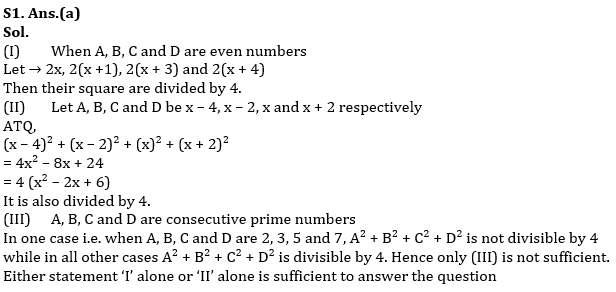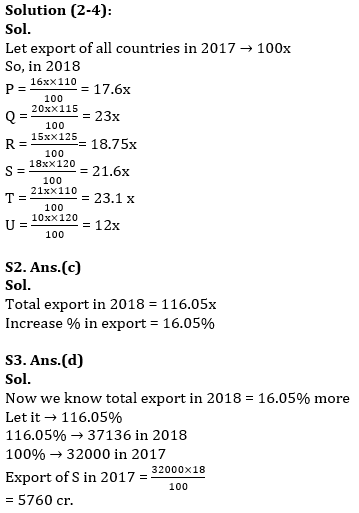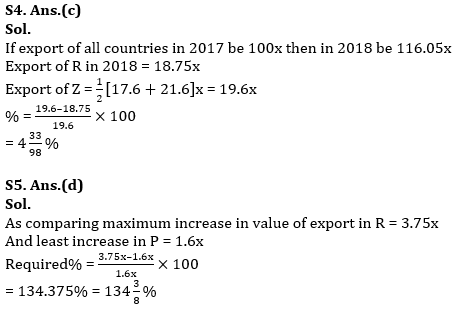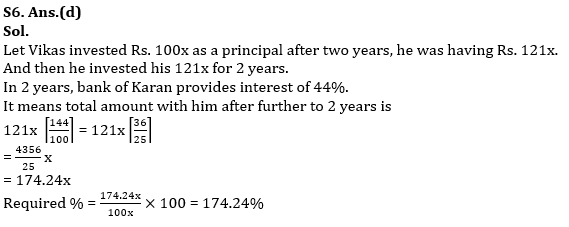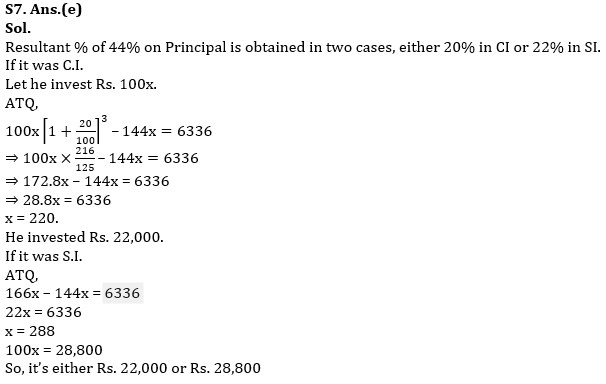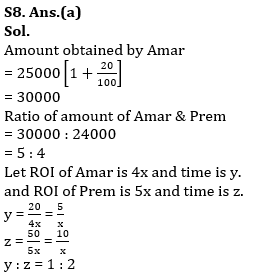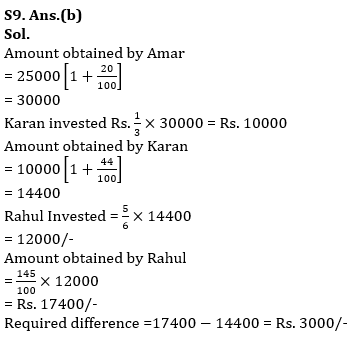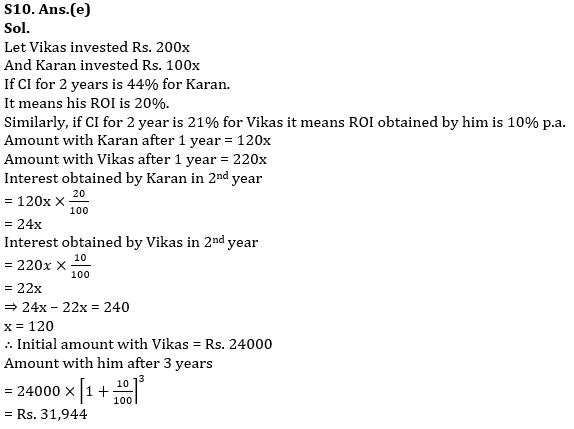#### Congratulations!Union Budget 2023-24: Free PDF